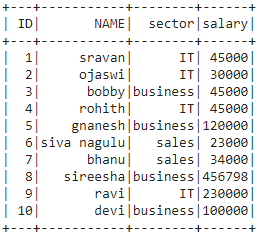# Count values by condition in PySpark Dataframe

• Last Updated : 16 Jul, 2021

In this article, we are going to count the value of the Pyspark dataframe columns by condition.

Creating Dataframe for demonstration:

Attention geek! Strengthen your foundations with the Python Programming Foundation Course and learn the basics.

To begin with, your interview preparations Enhance your Data Structures concepts with the Python DS Course. And to begin with your Machine Learning Journey, join the Machine Learning - Basic Level Course

## Python3

 `# importing module``import` `pyspark` `# importing sparksession from``# pyspark.sql module``from` `pyspark.sql ``import` `SparkSession` `# creating sparksession and giving an app name``spark ``=` `SparkSession.builder.appName(``'sparkdf'``).getOrCreate()` `# list  of employee data with 10 row values``data ``=` `[[``"1"``, ``"sravan"``, ``"IT"``, ``45000``],``        ``[``"2"``, ``"ojaswi"``, ``"IT"``, ``30000``],``        ``[``"3"``, ``"bobby"``, ``"business"``, ``45000``],``        ``[``"4"``, ``"rohith"``, ``"IT"``, ``45000``],``        ``[``"5"``, ``"gnanesh"``, ``"business"``, ``120000``],``        ``[``"6"``, ``"siva nagulu"``, ``"sales"``, ``23000``],``        ``[``"7"``, ``"bhanu"``, ``"sales"``, ``34000``],``        ``[``"8"``, ``"sireesha"``, ``"business"``, ``456798``],``        ``[``"9"``, ``"ravi"``, ``"IT"``, ``230000``],``        ``[``"10"``, ``"devi"``, ``"business"``, ``100000``],``        ``]` `# specify column names``columns ``=` `[``'ID'``, ``'NAME'``, ``'sector'``, ``'salary'``]` `# creating a dataframe from the lists of data``dataframe ``=` `spark.createDataFrame(data, columns)` `# display dataframe``dataframe.show()`

Output:### Method 1: Using select(), where(), count()

where(): where is used to return the dataframe based on the given condition by selecting the rows in the dataframe or by extracting the particular rows or columns from the dataframe. It can take a condition and returns the dataframe

Syntax: where(dataframe.column condition)

Where,

• Here dataframe is the input dataframe
• column is the column name where we have to raise a condition

count(): This function is used to return the number of values/rows in a dataframe

Syntax: dataframe.count()

Example 1: Python program to count values in NAME column where ID greater than 5

## Python3

 `# count values in NAME column``# where ID greater than 5``dataframe.select(``'NAME'``).where(dataframe.``ID``>``5``).count()`

Output:

`5`

Example 2: Python program to count values in all column count where ID greater than 3 and sector = IT

## Python3

 `# count values in all column count``# where ID greater than 3 and sector = IT``dataframe.select().where((dataframe.``ID``>``3``) &``                         ``(dataframe.sector``=``=``'IT'``)).count()`

Output:

`2`

### Method 2: Using filter(), count()

filter(): It is used to return the dataframe based on the given condition by removing the rows in the dataframe or by extracting the particular rows or columns from the dataframe. It can take a condition and returns the dataframe

Syntax: filter(dataframe.column condition)

Where,

• Here dataframe is the input dataframe
• column  is the column name where we have to raise a condition

Example 1: Python program to count ID column  where ID =4

## Python3

 `# count ID column  where ID =4``dataframe.select(``'ID'``).where(dataframe.``ID` `=``=` `4``).count()`

Output:

`1`

Example 2: Python program to count ID column  where ID > 4  and sector is sales or IT

## Python3

 `# count ID column  where ID > 4 ``# and sector is sales or IT``dataframe.select(``'ID'``).where((dataframe.``ID``>``4``) &``                             ``((dataframe.sector``=``=``'sales'``)|``                              ``(dataframe.sector``=``=``'IT'``))).count()`

Output:

`3`

My Personal Notes arrow_drop_up